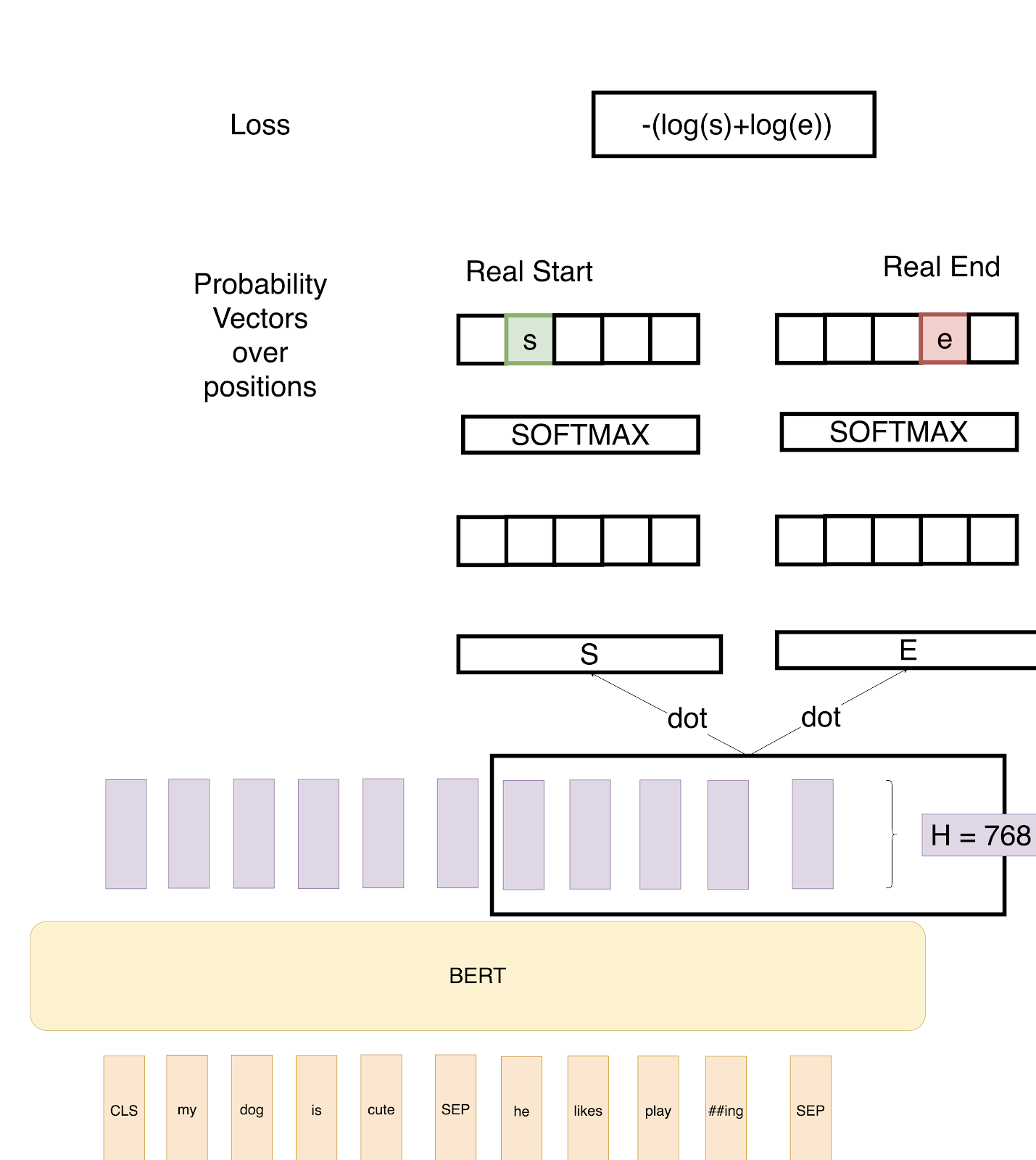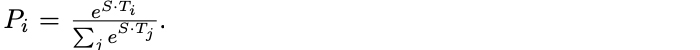### Using BERT and Hugging Face to Create a Question Answer Model

In a recent post on BERT, we discussed BERT transformers and how they work on a basic level. The article covers BERT architecture, training data, and training tasks.

However, we don’t really understand something before we implement it ourselves. So in this post, we will implement a Question Answering Neural Network using BERT and a Hugging Face Library.

In this task, we are given a question and a paragraph in which the answer lies to our BERT Architecture and the objective is to determine the start and end span for the answer in the paragraph.As explained in the previous post, in the above example we provide two inputs to the BERT architecture. The paragraph and the question are separated by the <SEP> token. The purple layers are the output of the BERT encoder.

We now define two vectors S and E (which will be learned during fine-tuning) both having shapes (1×768). We then take a dot product of these vectors with the second sentence’s output vectors from BERT, giving us some scores. We then apply Softmax over these scores to get probabilities. The training objective is the sum of the log-likelihoods of the correct start and end positions. Mathematically, for the Probability vector for Start positions:Where T_i is the word we are focusing on. An analogous formula is for End positions.

To predict a span, we get all the scores — S.T and E.T and get the best span as the span having the maximum Score, that is max(S.T_i + E.T_j) among all j≥i.

### How Do We Do This Using Hugging Face?

Hugging Face provides a pretty straightforward way to do this.

The output is:

```Question: How many pretrained models are available in Transformers?

Question: What do Transformers provide?

Question: Transformers provide interoperability between which frameworks?

Answer: TensorFlow 2. 0 and PyTorch```

So, here we just used the pretrained tokenizer and model on the SQuAD dataset provided by Hugging Face to get this done.

```tokenizer = AutoTokenizer.from_pretrained(“bert-large-uncased-whole-word-masking-finetuned-squad”)